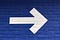# Best of Modern JavaScript — Arrow Function SyntaxPhoto by Matt Ridley on Unsplash

Since 2015, JavaScript has improved immensely.

It’s much more pleasant to use it now than ever.

In this article, we’ll look at arrow functions in JavaScript.

# Arrow Function Syntax

We can create arrow functions by using the following syntax:

`() => {  //... }`

or

`x => {  //...}`

or

`(x, y) => {  //... }`

If we have one parameter, then we don’t need the parentheses.

We can specify the body by writing:

`x => {  return x * x}`

or:

`x => x * x`

Arrow functions are great for reducing the verbosity of our code.

For example, we can reduce:

`const squares = [1, 2, 3].map(function (x) { return x * x });`

to:

`const squares = [1, 2, 3].map(x => x * x);`

# Omitting Parentheses Around Single Parameters

We can remove the parentheses if we have a single parameter that doesn’t have a default value.

For instance, we can write:

`[1, 2, 3].map(x => 4 * x)`

If we have anything else, we need the parentheses.

We can define default parameters by writing:

`[1, undefined, 3].map((x = 2) => 4 * x)`

# Propagating Variable Values

Variable values can be propagated statically or dynamically.

Static propagation is getting variable values from outside the function:

`const x = 'foo';function foo(y) {  return x;}`

Dynamic propagation is getting variable value from parameters:

`function bar(arg) {    return arg; // value received dynamically}`

Arrow functions don’t bind to their own special values.

These special values include:

• `arguments`
• `super`
• `this`
• `new.target`

# Arrow Functions Bind Loosely

Arrow functions bind loosely.

If we have:

`const foo = x => (x % 5) === 0 ? x : 0;`

Then `x => (x % 5) === 0` is considered to be part of the function.

To keep the whole expression together as the function, we can wrap them in the parentheses:

`const foo = x => ((x % 5) === 0 ? x : 0);`

We should wrap whatever we want in the function wither parentheses.

# No Line Break After Arrow Function Parameters

We can’t add a line break after the arrow function parameters.

For instance, we can’t write:

`const foo = (x, y) => {  return x + y;};`

We’ll get a syntax error if we write that.

Instead, we write:

`const foo = (x, y) => {  return x + y;};`

Then it’ll run.

This way, if our arrow function has only one parameter, we can omit the parentheses and still keep it valid.

# No Statements as Expression Bodies

Expressions are code that returns a value.

For instance:

`3 + 39foo(1)'abc'.length`

all return something and are expressions.

Statements do things. The following are examples of statements:

`if (true) {   //...}return 123;`

Most expressions can be used as statements, just that they don’t do anything.

We can put expressions into arrow functions if it’s one line long and the value will be returned.

For example, we can write:

`Promise.resolve(1).then(x => console.log(x));`

But we’ve to put statements in braces, so we’ve to write:

`Promise.resolve(1).then(x => {  throw x});`Photo by Nick Fewings on Unsplash

# Conclusion

Arrow functions can be defined and used in various ways.

Web developer. Subscribe to my email list now at https://thewebdev.info/subscribe/. Email me at hohanga@gmail.com

Web developer. Subscribe to my email list now at https://thewebdev.info/subscribe/. Email me at hohanga@gmail.com